# RD Sharma Solutions For Class 7 Maths Chapter 16 Congruence Exercise 16.4

Our expert team have designed the solutions for RD Sharma Solutions for Class 7 Maths Chapter 16 to help students prepare for their exams effortlessly.

RD Sharma Solutions for Class 7 is one of the best reference materials for CBSE students. Learners can download the pdf from the links provided below. The experts suggest students to practise the solutions many numbers of times and yield good results in their exams. In Exercise 16.4 of Chapter 16 Congruence, we shall discuss about the ASA congruence condition for two congruent triangles and their properties.

## Download the PDF of RD Sharma Solutions For Class 7 Maths Chapter 16 – Congruence Exercise 16.4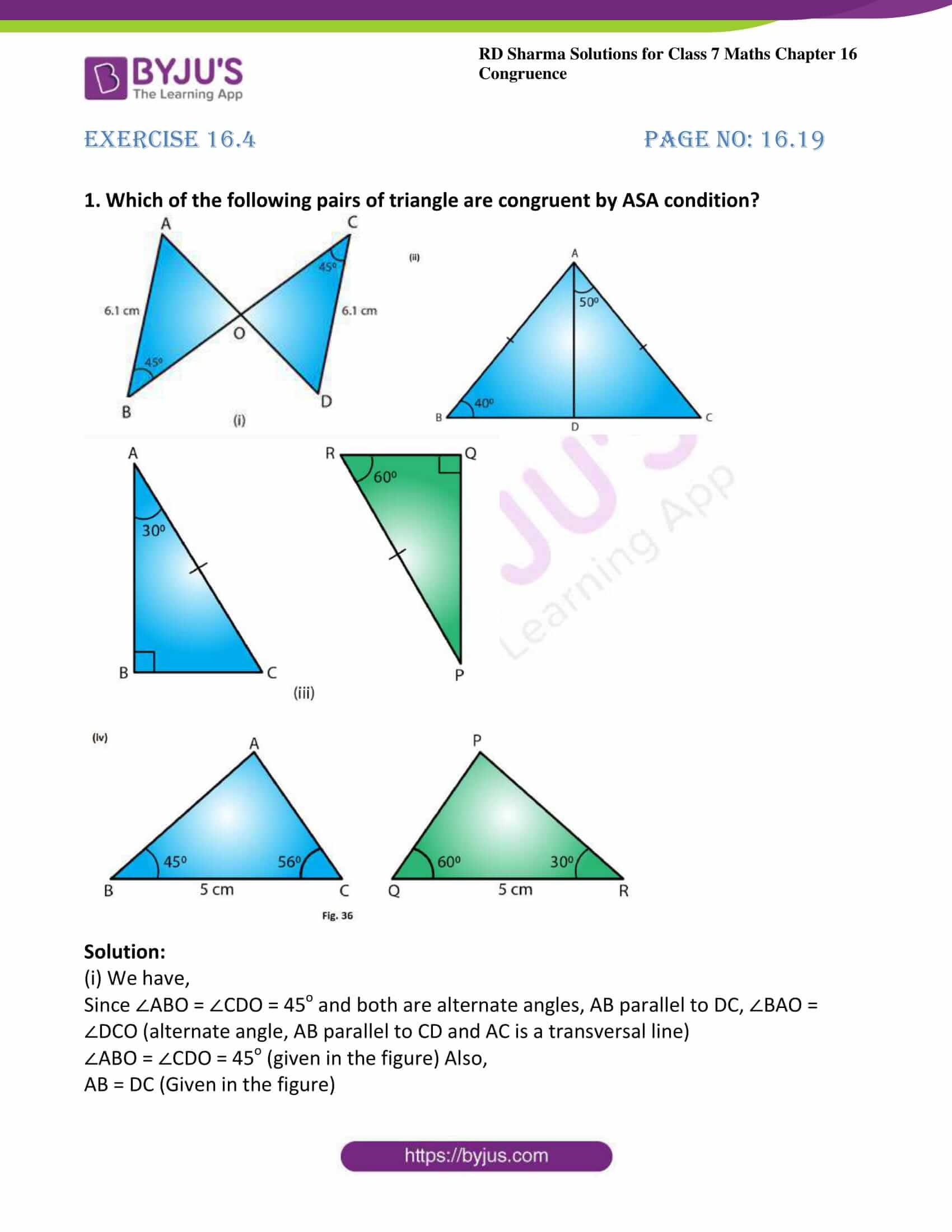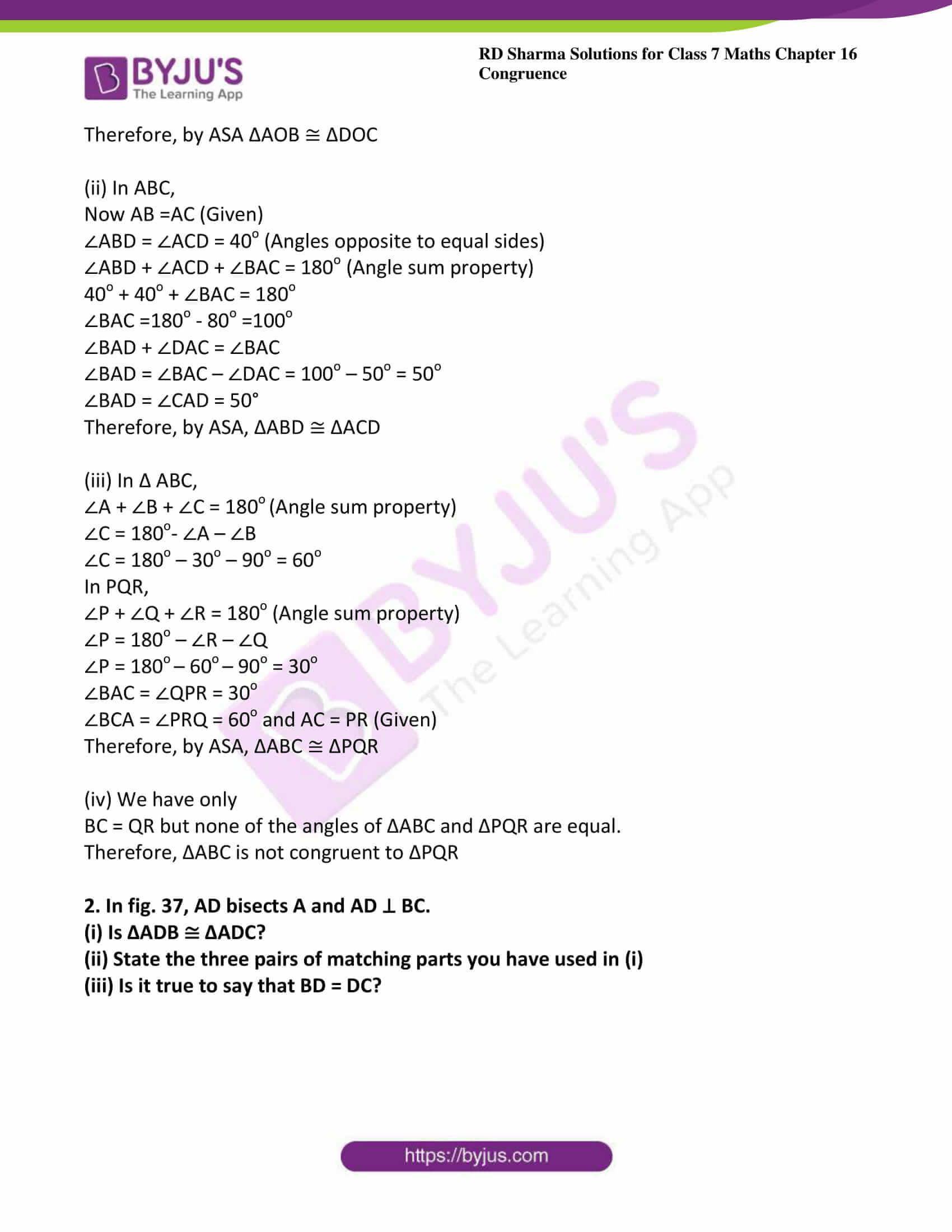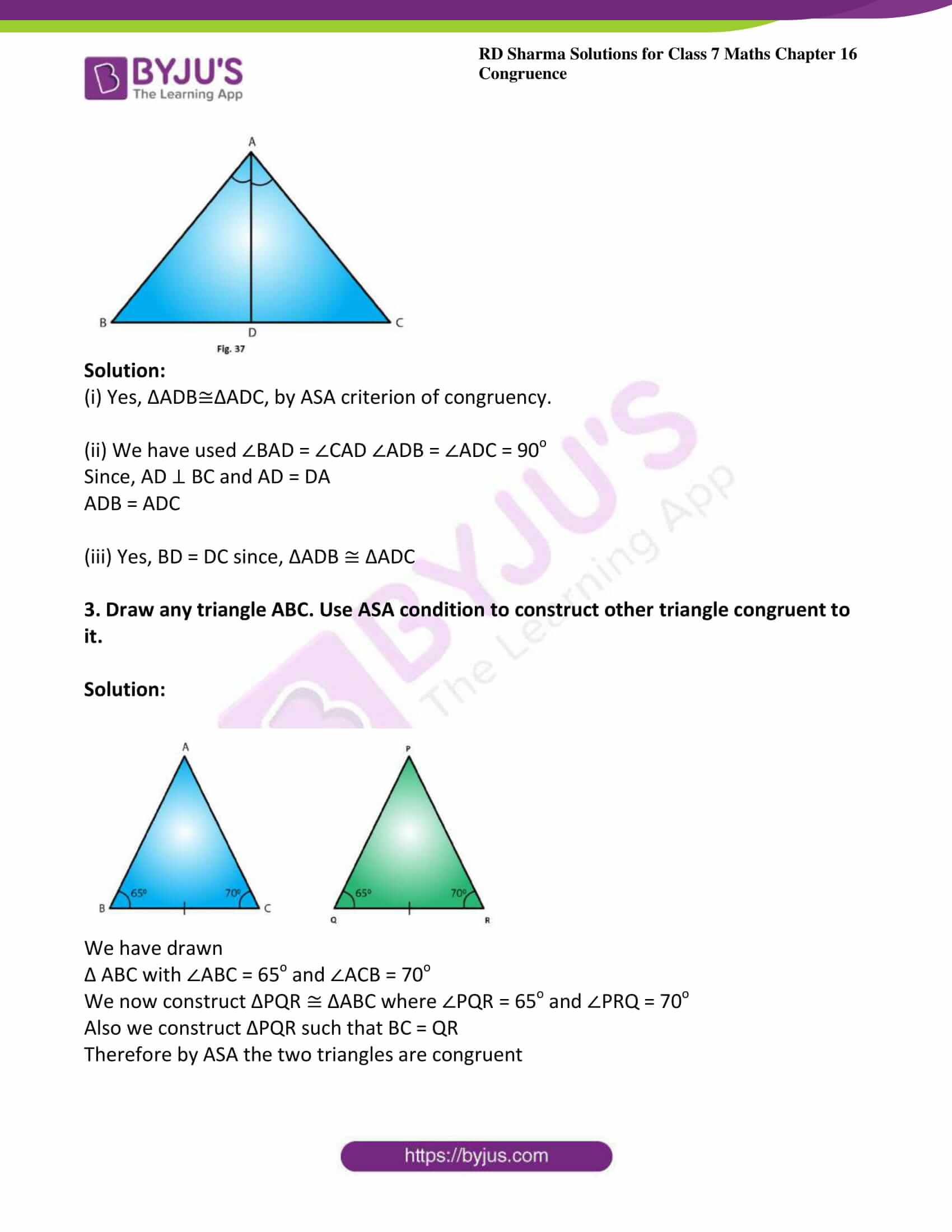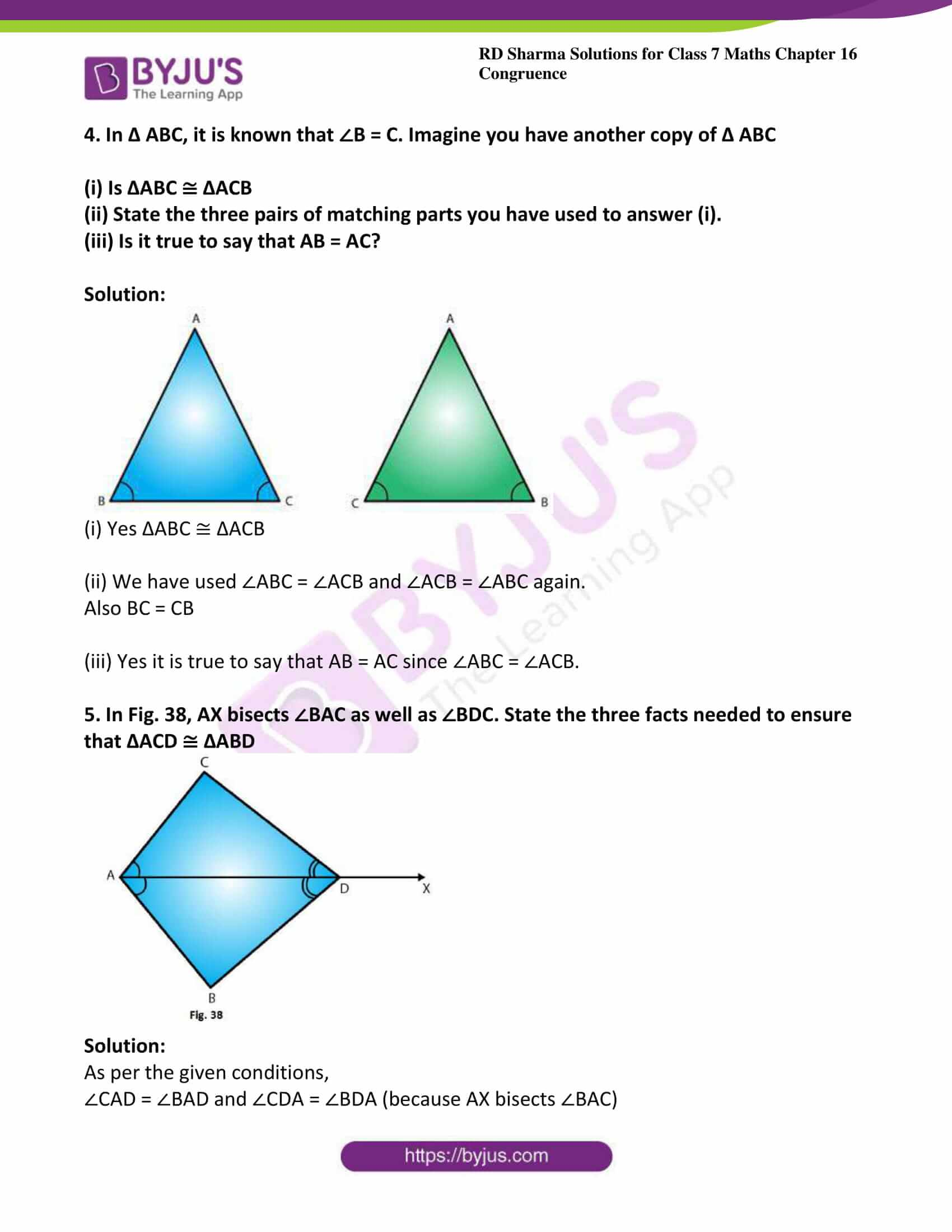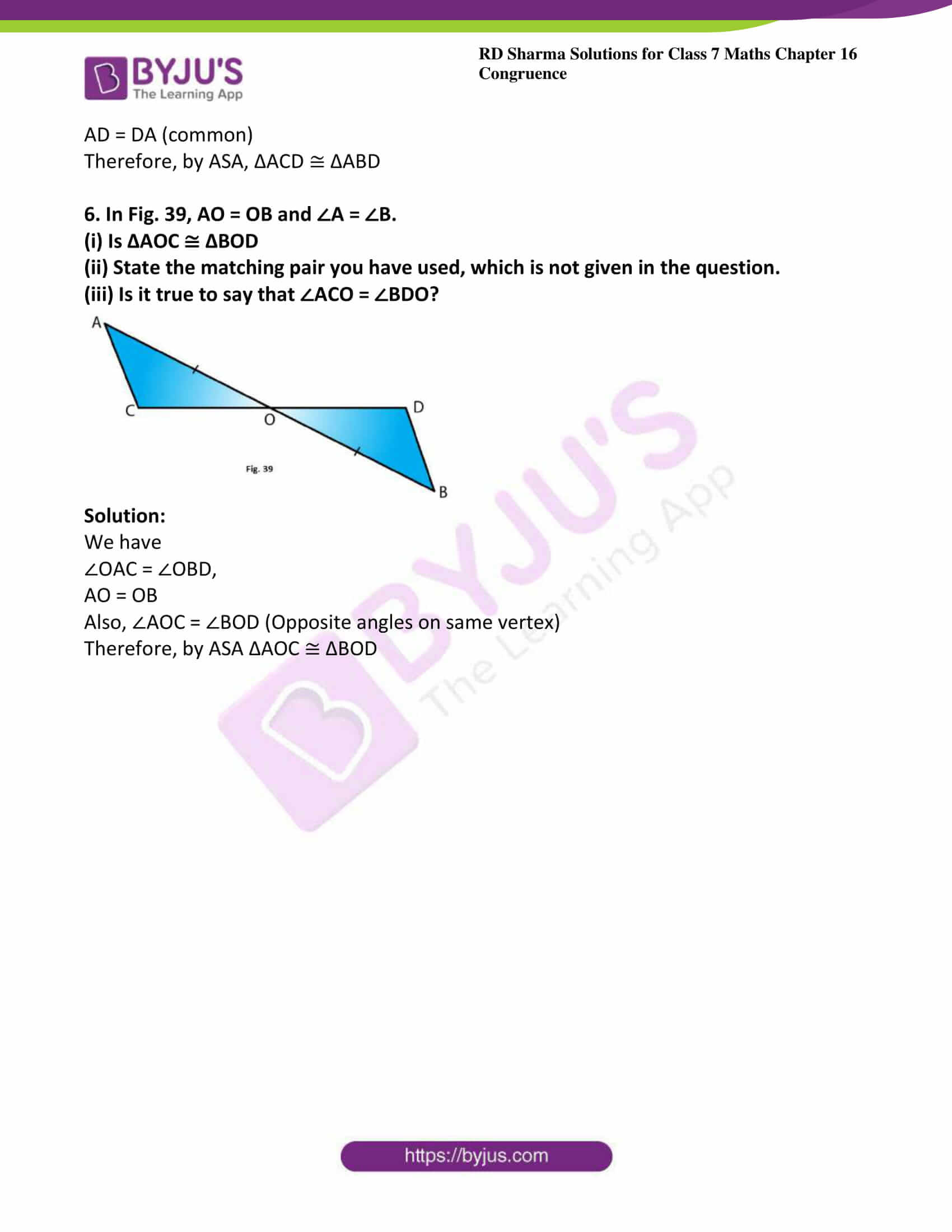### Access answers to Maths RD Sharma Solutions For Class 7 Chapter 16 – Congruence Exercise 16.4

Exercise 16.4 Page No: 16.19

1. Which of the following pairs of triangle are congruent by ASA condition?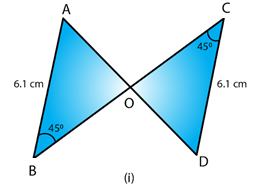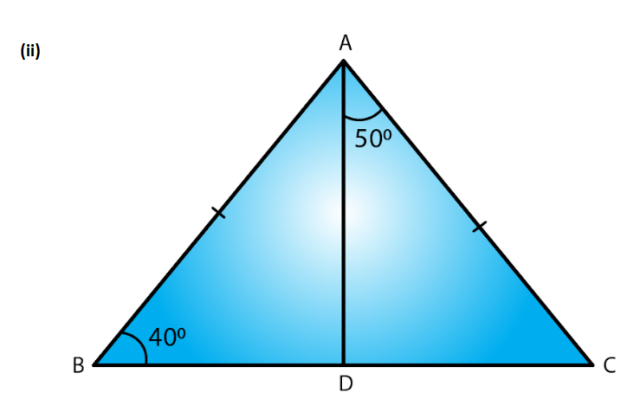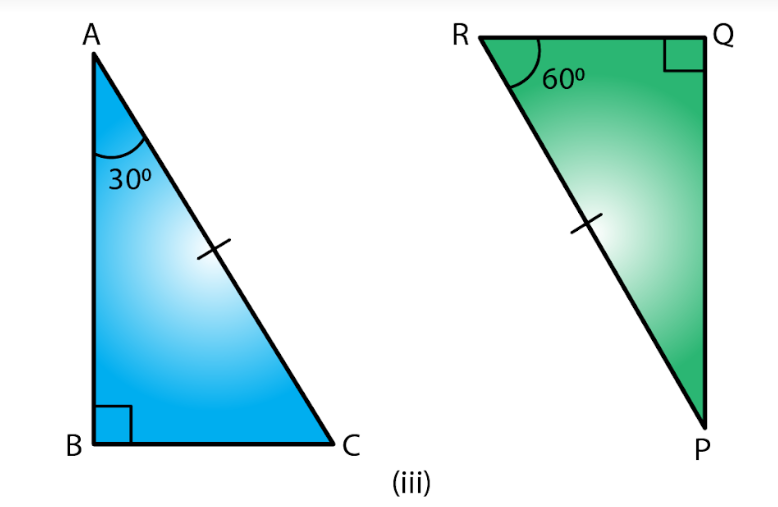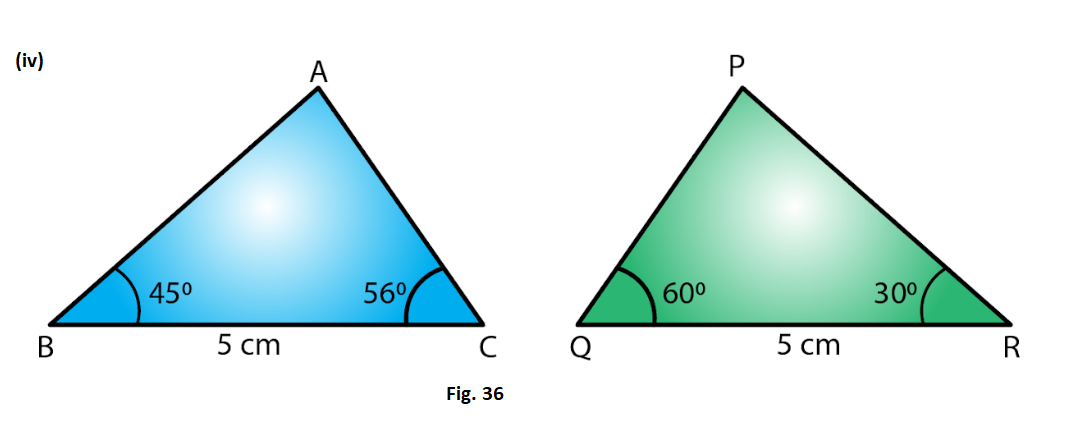Solution:

(i) We have,

Since ∠ABO = ∠CDO = 45o and both are alternate angles, AB parallel to DC, ∠BAO = ∠DCO (alternate angle, AB parallel to CD and AC is a transversal line)

∠ABO = ∠CDO = 45o (given in the figure) Also,

AB = DC (Given in the figure)

Therefore, by ASA ΔAOB ≅ ΔDOC

(ii) In ABC,

Now AB =AC (Given)

∠ABD = ∠ACD = 40o (Angles opposite to equal sides)

∠ABD + ∠ACD + ∠BAC = 180o (Angle sum property)

40o + 40o + ∠BAC = 180o

∠BAC =180o – 80o =100o

∠BAD = ∠BAC – ∠DAC = 100o – 50o = 50o

Therefore, by ASA, ΔABD ≅ ΔACD

(iii) In Δ ABC,

∠A + ∠B + ∠C = 180o (Angle sum property)

∠C = 180o– ∠A – ∠B

∠C = 180o – 30o – 90o = 60o

In PQR,

∠P + ∠Q + ∠R = 180o (Angle sum property)

∠P = 180o – ∠R – ∠Q

∠P = 180o – 60o – 90o = 30o

∠BAC = ∠QPR = 30o

∠BCA = ∠PRQ = 60o and AC = PR (Given)

Therefore, by ASA, ΔABC ≅ ΔPQR

(iv) We have only

BC = QR but none of the angles of ΔABC and ΔPQR are equal.

Therefore, ΔABC is not congruent to ΔPQR

(ii) State the three pairs of matching parts you have used in (i)

(iii) Is it true to say that BD = DC?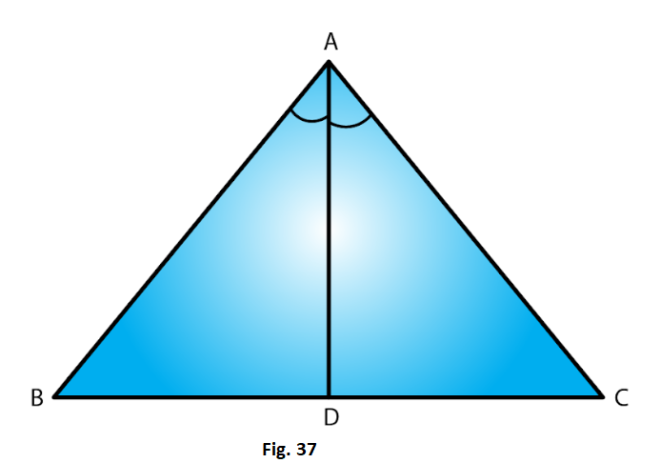Solution:

3. Draw any triangle ABC. Use ASA condition to construct other triangle congruent to it.

Solution: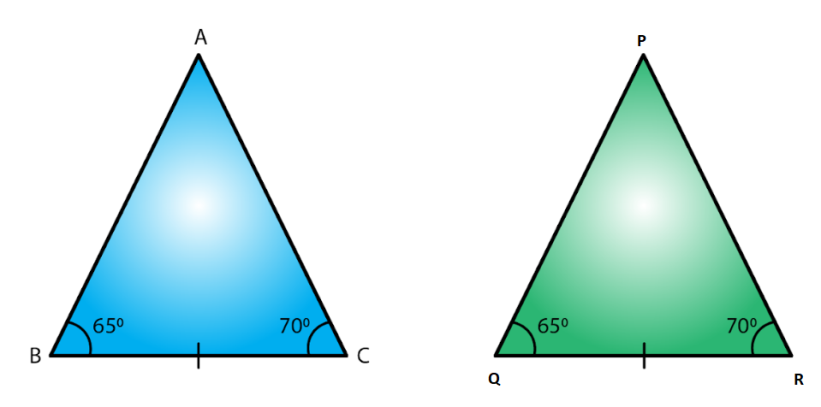We have drawn

Δ ABC with ∠ABC = 65o and ∠ACB = 70o

We now construct ΔPQR ≅ ΔABC where ∠PQR = 65o and ∠PRQ = 70o

Also we construct ΔPQR such that BC = QR

Therefore by ASA the two triangles are congruent

4. In Δ ABC, it is known that ∠B = C. Imagine you have another copy of Δ ABC

(i) Is ΔABC ≅ ΔACB

(ii) State the three pairs of matching parts you have used to answer (i).

(iii) Is it true to say that AB = AC?

Solution: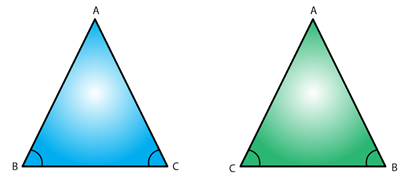(i) Yes ΔABC ≅ ΔACB

(ii) We have used ∠ABC = ∠ACB and ∠ACB = ∠ABC again.

Also BC = CB

(iii) Yes it is true to say that AB = AC since ∠ABC = ∠ACB.

5. In Fig. 38, AX bisects ∠BAC as well as ∠BDC. State the three facts needed to ensure that ΔACD ≅ ΔABD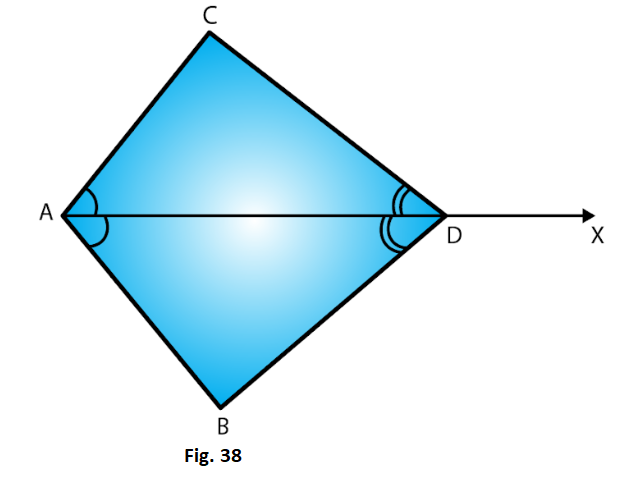Solution:

As per the given conditions,

Therefore, by ASA, ΔACD ≅ ΔABD

6. In Fig. 39, AO = OB and ∠A = ∠B.

(i) Is ΔAOC ≅ ΔBOD

(ii) State the matching pair you have used, which is not given in the question.

(iii) Is it true to say that ∠ACO = ∠BDO?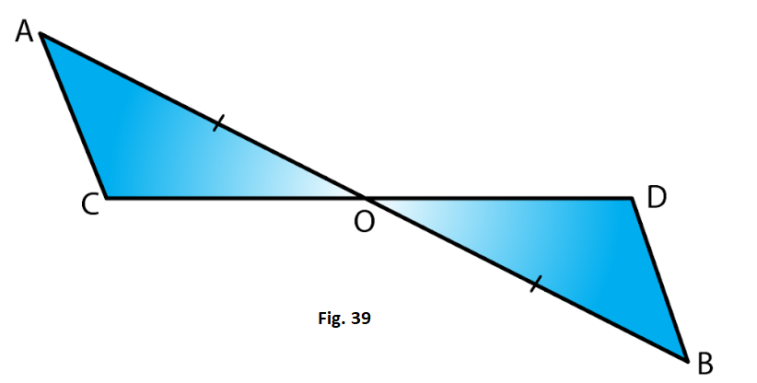Solution:

We have

∠OAC = ∠OBD,

AO = OB

Also, ∠AOC = ∠BOD (Opposite angles on same vertex)

Therefore, by ASA ΔAOC ≅ ΔBOD